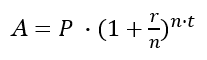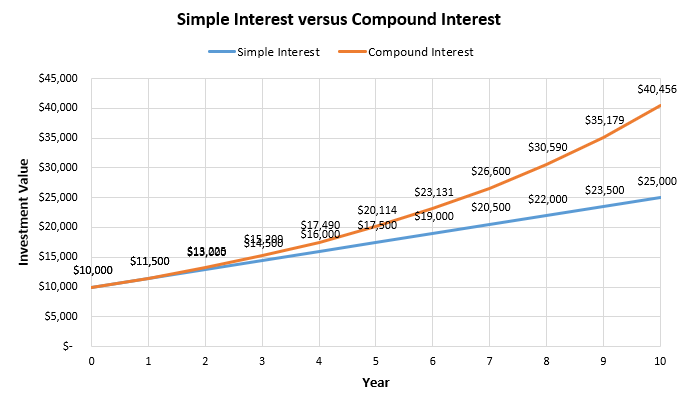# Compound Interest Calculator

Use this calculator to easily calculate the compound interest and the total future value of a deposit based on an initial principal. Allows adding money into the deposit, as well as calculating daily, monthly, quarterly, semiannual, and annual interest compounding, corresponding to compounding once per day, month, quarter, 6-months and 12-months (once per year).

\$
%
Share calculator:

Embed this tool:
get code

## What is compound interest

Compound interest is a type of interest in which the interest amount is periodically added to the principal amount and new interest is subsequently accrued over interest from past periods. It is a very powerful tool for increasing your capital and is a basic calculation related to personal savings plan or strategy, as well as long term growth of a mutual fund or a stock market portfolio. Compounding interest is the most basic example of capital reinvestment.

## Using the compound interest calculator

Our compound interest calculator is a versatile tool which will help you:

• calculate the final amount of money you will be able to save
• calculate how compounding increases your savings over time
• understand the difference between starting to save now or later

Start by entering your initial deposit or investment, or your current balance if you already have a deposit. This is used as a basis for all calculations. Then enter how long you want to keep the deposit or investment, usually in years, but we also support other time periods.

Enter the annual interest rate: this is usually listed as APR on offers and on bank product comparison sites and does not take compounding into account. This is different from the Annual Percentage Yield (APY) a.k.a. Effective Annual Interest Rate which our calculator will calculate for you, as it depends on the compounding period. Both the APR and APY do not account for fees and other expenses in servicing the deposit or investment.

Continue by specifying the compounding period - you may need to consult your bank on this detail, but it is often disclosed on offers and certificate of deposit (CD) descriptions. The tool supports:

• annual compounding
• semiannual compounding
• quarterly compounding
• monthly compounding
• daily compounding

Finally, if you plan on making regular contributions — adding to the deposit on a regular basis (monthly, yearly, etc.) — enter the amount and the period on which you will make it, as well as whether you will make it in the beginning or the end of the period.

The interest calculator will output: the value of your deposit or investment at the end of the period, the interest accrued, the effective interest rate, the total amount of additional deposits made and the percentage capital growth.

## Compound interest formula

The compound interest formula is:where A is the Accrued amount (principal plus interest), P is the principal, r is the Annual interest rate (not compounded, not APY) in decimal, t is the time in years, and n is the number of compounding periods per unit t.

The formula for the effective interest rate is:where I is the effective interest rate and the rest of the notation is as above. These formulas can be spun accordingly to solve for principal and time. If you wonder how to calculate compound interest, these formulas provide the answer.

## Effect of the compounding period

The compounding frequency, which is the time period at which interest is added to the principal, can have a slight positive effect on the effective interest rate versus the nominal annual interest rate. Using shorter compounding periods in our compound interest calculator will easily show you how big that effect is. You get the best effective rate when you have daily compounding (also called continuous compounding) and slightly worse with monthly or yearly compounding.

## Compound interest calculation example

In this example the task is to estimate the accrued interest, the total accrued interest, and the capital growth percentage of a certificate of deposit with an initial value of \$10,000 and an annual interest rate of 2% over a period of three years. There will be no contributions (monthly or yearly deposits) to keep the calculation simpler.

During the first year the math is just that of simple interest. Starting with \$10,000 at 2% interest results in \$10,000 x 0.02 = \$200 interest for a final sum at the end of year one of \$10,200. The rate of capital growth is simply the interest rate. In year two we start compounding by adding the \$200 in interest to the principal thus starting year two with \$10,200. The interest on that is now not \$200 like in year one, but \$10,200 x 0.02 = \$204, hence at the end of year two the deposit will be worth \$10,404. The capital growth rate is a straightforward percentage increase calculation: \$10,404 / \$10,000 = 4.04%.

The table below shows the result of applying these equations to years 3, 4, and 5, and the final result:

Compound interest example table with 5 years of yearly compounding
YearStarting ValueAccrued InterestFinal ValueTotal InterestCapital Growth
1 \$10,000.00 \$200.00 \$10,200.00 \$200.00 2.000%
2 \$10,200.00 \$204.00 \$10,404.00 \$404.00 4.040%
3 \$10,404.00 \$208.08 \$10,612.08 \$612.08 6.121%
4 \$10,612.08 \$212.24 \$10,824.32 \$824.32 8.243%
5 \$10,824.32 \$216.49 \$11,040.81 \$1,040.81 10.408%

The final value after 5 years is \$11,041 whereas with simple interest it would have been just \$11,000. This might not seem like much, but if the rate of return is higher or the period over which compounding occurs is longer, the compounding effect can be dramatic.

## Simple Interest versus Compound Interest

The above example has already shown the difference between simple versus compound interest. To make it more pronounced, let us examine a hypothetical investment with a 15% annual rate of return over ten years. Assuming the returns can be reinvested at the same rate at the end of each year, note how the difference increases as the number of compounding periods goes up.At year five the gap in return is more than \$2,500 while at year ten it is over \$15,000 on that same \$10,000 initial investment. That's a capital growth of 405% compared to just 250%. That is the power of compounding. For a deeper exploration of the topic, consider reading our article on how compounding works with investments.

## Financial caution

This is a simple online tool which is a good starting point in estimating the return on investment and capital growth you can expect from a bank deposit or a similar investment, but is by no means the end of such a process. You should always consult a qualified professional when making important financial decisions and long-term agreements, such as long-term bank deposits. Use the information provided by the software critically and at your own risk.

#### Cite this calculator & page

If you'd like to cite this online calculator resource and information as provided on the page, you can use the following citation:
Georgiev G.Z., "Compound Interest Calculator", [online] Available at: https://www.gigacalculator.com/calculators/compound-interest-calculator.php URL [Accessed Date: 27 Mar, 2023].